# Deriving the Ampère-Maxwell law

Maxwell’s equations tell us how electricity and magnetism are related to each other. After deriving the first two fundamental laws, namely Gauss’s law of electricity and Gauss’s law of magnetism, we are now ready to look at a more complicated, yet equally important law known as the Ampère-Maxwell law. In this article, we derive the differential equation associated with this law, and briefly discuss its physical implications.

# Deriving the differential equation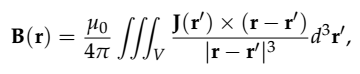Equation 1

The expression in the integrand can be simplified if we take note of the following relation

Allowing us to re-write Eq(1) as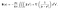Equation 2

At this point, we can use the vector identity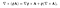Equation 3

and set

to write

However, we note that

Because the Nabla operator is in term of r coordinates only rather than r’. Therefore we arrive at

which ultimately leads to the modified expression for the magnetic field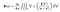Equation 4: Biot-Savart law in terms of current density J

At this stage, seeing that curl operator inside the volume integral makes us think of what would happen if we apply another curl operator to both sides of Eq(4). For instance, using the double-curl identity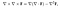Equation 5: double-curl vector identity

we can write Eq(4) as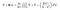Equation 6

where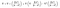Equation 7

We thus write

where we have used the identity

and also the fact that the Laplacian operator is in terms of r and not r’. The next term in the expansion of Eq(7) becomes

This leads to

The 2nd and 4th terms here vanish because J(r’) is independent of r, so

this leaves us with just

from here on we expand

but

from basic vector identities. Furthermore, we can prove that

which leads to

Hence, we write

This leads to the expression for the curl of the magnetic field

or simply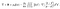Equation 8: curl of the magnetic field

If we assume a steady-state magnetic current, the 2nd term in Eq(8) can be neglected altogether, which leads to Ampère’s law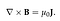Equation 9: Ampère’s law in differential form

Now, suppose we take a dot product of both sides with a surface area element dS and integrate over an entire enclosed surface, then

the term on the right is nothing more than the total electric current enclosed in the surface so

At this point, we can apply Stokes’ theorem to simplify the double integral on the left and arrive at the integral form of Ampère’s law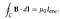Equation 10: Ampère’s law in integral form

What both Eq(9) and Eq(10) tell us is that a circulating magnetic field gives rise to a current density in the direction perpendicular to that circulation, or vice-versa: an electric current travelling in a specific direction gives rise to a circulating magnetic field perpendicular to it. This is the mathematical equivalent of what is known as the “right-hand rule” of electromagnetism. This fundamental equation allows us to explain why wires carrying electric currents placed parallel to each other exert forces on each other (these forces being proportional to the magnetic field around them).

One problem with Ampère’s law as we have stated it though is that it implies the following result

Because

must be true for any vector field F. This would imply that

which violates the law of charge conservation (violating conservation laws is a general no-no in physics). So what do we do? Does that mean that our law is incorrect? There is in fact a quick fix to this: we start with the continuity equation for electric charge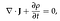Equation 11: continuity equation of electric charge

where 𝞺 denotes the charge density as a function of space. Now, we can use Gauss’s law of electric fields in a slightly modified way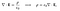Equation 12: Gauss’s law of electric fields

which allows us to write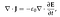Equation 13

Next, we would like this to be consistent with the result

so we add this term in Eq(13) to Eq(9) in order to obtain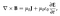Equation 14: the Ampère-Maxwell law

which gives us what is known as the Ampère-Maxwell law. This law has the same physical implications we just discussed with (namely the right-hand rule and that electric currents give rise to circulating magnetic fields) but it fixes the charge conservation problem that we found in Ampère’s law.

Oscar is a physicist, educator and STEM enthusiast. He is currently finishing a PhD in Theoretical Physics with a focus on photonics and stochastic dynamics.

## More from Oscar Nieves

Oscar is a physicist, educator and STEM enthusiast. He is currently finishing a PhD in Theoretical Physics with a focus on photonics and stochastic dynamics.